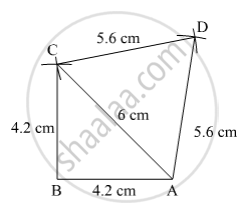SSC (English Medium) Class 8Maharashtra State Board
Share

# Construct ☐ Barc Such that L(Ba) = L(Bc) = 4.2 Cm, L(Ac) = 6.0 Cm, L(Ar) = L(Cr) = 5.6 Cm - SSC (English Medium) Class 8 - Mathematics

ConceptKinds of Quadrilaterals Concept of Parallelogram

#### Question

Construct ☐ BARC such that l(BA) = l(BC) = 4.2 cm, l(AC) = 6.0 cm, l(AR) = l(CR) = 5.6 cm

#### Solution

Steps of Construction:

Step 1:
Draw BA = 4.2 cm.

Step 2: With B as centre and radius 4.2 cm, draw an arc.

Step 3: With A as centre and radius 6 cm, draw an arc cutting the previous arc at C.

Step 4: Join BC.

Step 5: With A as centre and radius 5.6 cm, draw an arc.

Step 6: With C as centre and radius 5.6 cm, draw an arc cutting the previous arc at R.

Step 7: Join AR and CR.Here, BARC is the required quadrilateral.

Is there an error in this question or solution?

#### APPEARS IN

Balbharati Solution for Balbharati Class 8 Mathematics (2019 to Current)
Chapter 8: Quadrilateral : Constructions and Types
Practice Set 8.3 | Q: 5 | Page no. 50
Solution Construct ☐ Barc Such that L(Ba) = L(Bc) = 4.2 Cm, L(Ac) = 6.0 Cm, L(Ar) = L(Cr) = 5.6 Cm Concept: Kinds of Quadrilaterals - Concept of Parallelogram.
S